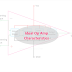The most important Ideal Op Amp characteristics are,
• Infinite Common Mode Rejection Ratio(CMRR)
• Infinite Output Voltage Range
• Infinite Voltage Gain
• Infinite High Input Impedance
• Zero Output Impedance
• Zero input voltage offset
• Infinite Power Supply Rejection Ratio
• Infinity Slew Rate
• Infinity bandwidth with zero phase shift
• Zero Output resistance
• Infinity Output Current Range
• Zero Noise or Distortion
• Infinite Open Loop Gain
• Infinity Voltage Amplification
• Low Output Resistance
• High Input Resistance
• Low Input Current

The Op-Amp or operational amplifier is a very popular amplifier used for so many useful applications. It generally comes in the form of integrated circuits or ICs. The operational amplifier can perform mathematical operations such as addition, subtraction, multiplication, division, differentiation, integration, etc. Basically, it works like a differential voltage amplifier. There is a major difference between an ideal operational amplifier and a practical operational amplifier. Because the actual characteristics cannot be obtained from a practical operation amplifier due to its internal power loss and other distortion factors. But you must know the actual characteristics of ideal OpAmp or operational amplifier.

The most common and important characteristic of an ideal opamp is high input impedance. The high input impedance implies that the operational amplifier does not consume any current(ideally) or consume a very low current(practically). If the input impedance is low then it will consume a very large current and will create a disturbance to the input circuit. So it is good to have a high input impedance that is why the opamp is designed with high input impedance. You can consider impedance for alternating and resistance for direct current.

Another property is the zero or low output impedance. The ideal opamp is designed with zero output impedance although it is not possible practically. If the output impedance is low the output circuit will work with maximum load current. The other advantage of the low impedance is, the voltage drop will be low so the maximum voltage will transfer from the OpAmp to the external load. When the maximum voltage has transferred the efficiency of the whole circuit will be increased. When the opamp works in a linear region the input voltage and output voltage change linearly. So, at the linear region, the operational amplifier gives the best output.

Common Mode Rejection Ratio(CMRR) - It is a very important characteristic. It ensures that the operational amplifier will reject the same part of the two input signals. It only responds when there is a difference in the positive and negative voltage applied to the input of the opamp. A high CMRR is required when a differential signal is needed to be amplified completely.

The infinity bandwidth property implies that the ideal opamp can work with any range of frequency with the same differential gain. The differential gain will not change with the changing of frequency of the input signal. It comes into use when high-frequency radio signals are needed to be amplified or recreated.

The concept of an infinite output current range in an ideal op amp means that it is capable of delivering any amount of current required by the load connected to its output without limitation. This implies that the op-amp can drive any load impedance without being affected by its current requirements. The reasoning behind this ideal characteristic is to simplify circuit analysis and design. By assuming an infinite output current range, it eliminates the need to consider current limitations and allows for more straightforward calculations and modeling of the op amp's behavior.

Ideal Op Amp Characteristics | Operational AmplifierReviewed by Author on December 11, 2021 Rating: 5